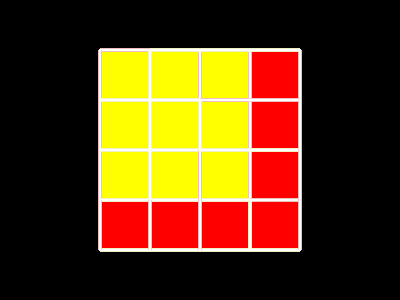# aUCBLogo Demos and Tests / dotgame;;; Connect-the-dots game  by Brian Harvey

be dotgame [size 5][player1 "Human][player2 "computer]
; Connect-the-dots game.  Input is the number of dots on each side.

local [offset lines computer person numboxes boxlists

oldmove boxes]

;   allFullScreen
;   (splitScreen 0.9)

hT

setScreenColor 0

cS
setPC
7

colors={6 4}

setPenSize [5 5]

offset=(size-1)*s/2

PU setPos List -offset -offset
board size
lines
=Se (crossmap [List (List ?1 ?2) (List ?1 1+?2)]
(
iSeq size-1) (iSeq size-2))
(
crossmap [List (List ?1 ?2) (List 1+?1 ?2)]
(
iSeq size-2) (iSeq size-1))

score={0 0}

numboxes=(size-1)*(size-1)

boxlists=(Array 5 0)

oldmove=[]

for [1 4][boxlists.i=[]]

boxlists.0=(crossmap [List ?1 ?2] (iSeq size-2) (iSeq size-2))

boxes=(Array size-1 0)

for [size-2] [boxes.i=(Array size-1 0)]

catch "WIN   ; play the game!

[   forever

[   amove player1

amove player2

if Key? [break]

]

]

if not emptyP oldmove ; make the last move white

[   setPC 7

PU
setPos
(first oldmove)*s-offset

PD
setPos
last oldmove)*s-offset

]

if score.1 score.2

[   print (Se player1 "player1 "lost score.1 "to score.2)

]

if score.1 score.2

[   print (Se player1 "player1 "won score.1 "to score.2)

]

if score.1 == score.2 [print [tie game]]

setPenSize [1 1]

notFullScreen

splitScreen

; --------------- Initial board display -------------------------

be board num

repeat num [dots num]

updateGraph

end

be dots num

PD

repeat num [setFC PC fillCircle PU rt 90 fd lt 90 PD]

PU lt 90 fd s*num rt 90 fd s

end

be amove nr player

ifElse player=="human

[   personmove nr

][   commove nr

]

updateGraph

end

; -------------- Human player's move ---------------------

be personmove nr

; Read a mouse click, turn it into a move if legal.

local [move direction found]

move=gmove

if Key? [stop]

if not legalp move

[   print [not a legal move!  Try again.]

personmove nr stop

]

drawline move nr
direction
=reverse (last move)-first move
found
=false

fillboxes nr move direction &found

if found [personmove nr]

end

be gmove

while [and  (MouseButtons == 0)  not Key?]

[   dispatchMessages

waitMS 100

]

while [and  (MouseButtons != 0)  not Key?]

[   dispatchMessages

waitMS 100

]

output findline (Int MousePos)+offset

end

be findline pos_

; Find the nearest vertical or horizontal line to the mouse click.

local [xrem yrem xpos ypos d]

d=1

xrem=Remainder (first pos_)+d s
yrem
=Remainder (first bF pos_)+d s
xpos
=(first pos_)+d-xrem
ypos
=(first bF pos_)+d-yrem

if xrem yrem

[   output List (List xpos/s ypos/s) (List xpos/s+ypos/s)

]

output List (List xpos/s ypos/s) (List xpos/s ypos/s+1)

end

be legalp move

; Output true if this is an undrawn line segment connecting two dots.

output MemberP move lines

end

; ----------------- Computer's move ----------------------

be commove nr

; The computer chooses a move, does the housekeeping for it.
; Strategy complete boxes if possible, otherwise pick a move that doesn't
; let the opponent complete a box.

local [move goodlines cohorts direction found l i]

ifElse not emptyP (Item boxlists)

[   move=lastline first (Item boxlists)
][

;         goodlines=filter "lineokay? lines

goodlines=[]

i=Int Max (count lines)*0.1 100    ;should be fairly big for good computer opponent

while [empty? goodlines]

[   l=pick lines

if LineOkay? l [push "goodlines break]

if i==[break]

i-=1

]

ifElse not emptyP goodlines

[   move=pick goodlines

][   cohorts=[]

makecohorts lines &cohorts
move
=lastline first smallest cohorts

]
]

drawline move nr
direction
=reverse (last move)-first move
found
=false

fillboxes nr move direction &found

if found [commove nr]

end

be lineokay? move

; Output true if this move won't let the opponent complete a box.

local [direction]

direction=reverse (last move)-first move

output and boxokay? first move
boxokay?
(first move)-direction

end

be boxokay? :box

; Output true if this box has fewer than 2 edges already drawn.

if or ((first :box) < 0) ((last :box) < 0) [output "true]

if or ((first :box) > (size-2)) ((last :box) > (size-2)) [output "true]

local [count_]

count_= (boxes.first :box).last :box

if emptyP count_ [count_=0]

output count_<2

end

be lastline :box

; :box has three lines drawn; find the missing one for us to draw.

if MemberP List :box :box+[0 1lines

[   output List :box :box+[0 1]

]

if MemberP List :box :box+[1 0lines

[   output List :box :box+[1 0]

]

if MemberP List :box+[0 1:box+[1 1lines

[   output List :box+[0 1:box+[1 1]

]

if MemberP List :box+[1 0:box+[1 1lines

[   output List :box+[1 0:box+[1 1]

]

output []   ; :box was full already (from makecohort)

end

be makecohorts lines &cohorts

; Partition the available boxes into chains, to look for the smallest.
; Note, the partition is not necessarily optimal -- this algorithm needs work.
; It's important that LINES be a local variable here, so that we can "draw"
; lines hypothetically that we're not really going to draw on the board.

local [cohort]

while [not emptyP lines]

[   cohort=[]

makecohort first lines &cohort &lines

push "cohorts cohort

]

end

be makecohort line_ &cohort &lines

; Group all the boxes in a chain that starts with this line.
; Mark the line as drawn (locally to caller), then look in both directions
; for completable boxes.

local [direction]

make "lines remove line_ lines
direction
=reverse (last line_)-first line_
makecohort1
(first line_)-direction &cohort &lines
makecohort1
first line_ &cohort &lines

end

be makecohort1 ::box &cohort &::lines

; Examine one of the boxes adjoining the line just hypothetically drawn.
; It has 0, 1, or 2 undrawn sides.  (If 3 or 4, wouldn't have gotten here.)
; 0 sides -> count the :box if not already, but no further lines in the chain.
; 1 side -> count the :box, continue the chain with its last side.
; 2 sides -> the :box isn't ready to complete, so it's not in this chain.

if or ((first ::box) < 0) ((last ::box) < 0) [stop]

if or ((first ::box) > (size-2)) ((last ::box) > (size-2)) [stop]

local [togo]

togo=filter [MemberP (List ::box+first ?  ::box+last ?::lines]
[[[
0 0] [0 1]] [[0 0] [1 0]]
[[
1 0] [1 1]] [[0 1] [1 1]]]

if (count togo)==[stop]

if not MemberP ::box cohort [push "cohort ::box]

if emptyP togo [stop]

local [line_]

line_List ::box+first first togo
::box
last first togo
makecohort line_
&cohort &::lines

end

be smallest cohorts [sofar []] [minsize dotgame::numboxes+1]

if emptyP cohorts [output sofar]

if (count first cohorts) < minsize

[   output (smallest bF cohorts first cohorts count first cohorts)

]

output (smallest bF cohorts sofar minsize)

end

; ----------- Common procedures for person and computer moves --------

be drawline move nr

; Actually draw the selected move on the screen.

if not emptyP oldmove

[   setPC 7

PU
setPos
(first oldmove)*s-offset

PD
setPos
last oldmove)*s-offset

]

setPC colors.nr

PU
setPos
(first move)*s-offset

PD
setPos
last move)*s-offset
oldmove
=move

end

be fillboxes nr move direction &found

; Implicit inputs (inherited from caller)
;   move is the move someone just made.
;   direction is [1 0] for vertical move, [0 1] for horizontal.
; Note that the line is drawn, check the two boxes (maybe) on either side,
; color them and count them for the appropriate player, see if game over.

lines=remove move lines

if boxbefore? move direction [fillbox (first move)-direction nr &found]

if boxafter?  move           [fillbox  first move            nr &found]

testwin

end

be boxafter? move

; Output true if the :box above or to the right of the move is now complete.

output (increment first move)==4

end

be boxbefore? move direction

; Output true if the :box below or to the left of the move is now complete.

local [p3]

p3=(first move)-direction

output (increment p3)==4

end

be increment :box

; If this isn't a :box at all (might be if the move was on a border),
; just output [].  Otherwise, increment the number in the boxes array,
; and move this :box from one of the boxlists to the next higher one.
; Output the new count of number of lines drawn in this :box.

if or ((first :box) < 0) ((last :box) < 0) [output []]

if or ((first :box) > (size-2)) ((last :box) > (size-2)) [output []]

local [count_]

count_=(boxes.first :box).last :box

if empty? count_ [count_=0]

setItem (last :boxboxes.first :box  count_+1

setItem count_ boxlists (remove :box boxlists.count_)

setItem count_+boxlists (fPut :box boxlists.(count_+1))

output count_+1

end

be fillbox :box nr &found

; Color in a completed :box, increase the :box count of its owner, and
; flag that a box was completed.

PU
setPos
:box*s-offset+PenSize/2+1

setFC colors.nr

fillRect [0 0] (List s s)-PenSize-2

updateGraph

score.nr=score.nr+1

found=true

end

; ------------------- Endgame processing --------------------

be testwin

if score.1+score.2 == numboxes [throw "win]

end
end